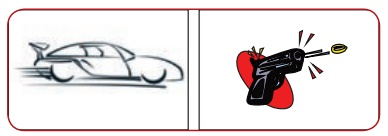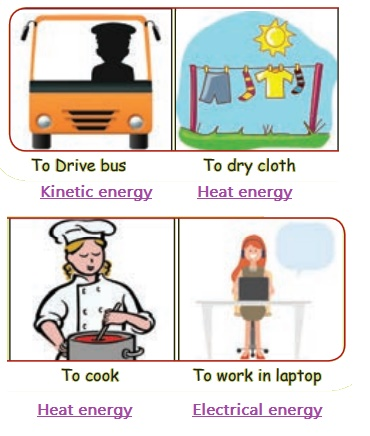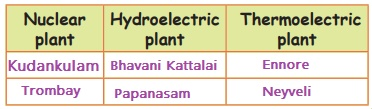Home | | Science 5th Std | Questions with Answers

## Chapter: 5th Science : Term 1 Unit 3 : Energy

5th Science : Term 1 Unit 3 : Energy : Text Book Back Exercises Questions with Answers, Solution, Evaluation; Choose the correct answer, Fill in the blanks, Say True or False, Match the following, Short Answers, Answer briefly, Answer in detail, Questions based on higher order thinking, Student Activities, Student Project

Energy ( Term 1 Chapter 3 | 5th Science)

Evaluation

1. When diesel is burnt chemical energy is converted into ________

a) wind energy

b) heat energy

c) solar energy

d) sound energy

2. Running water possesses ______

a) potential energy

b) chemical energy

c) kinetic energy

d) sound energy

3. Unit of energy is ________

a) kilo gram

b) newton

c) kelvin

d) joule

4. Which one of the following requires wind energy?

a) Bicycle

b) Photosynthesis

c) Parachute

d) Automobiles

5. Cow dung possesses ______

a) kinetic energy

b) chemical energy

c) solar energy

d) heat energy

II. Find out the energy conversion that takes place in the following.

1. Iron box : Chemical energy → Heat energy

2. Electric Iron box : Electrical energy Heat energy

3. Electric fan : Electrical energy → Mechanical energy

4. Speaker : Electrical energy Sound energy

5. Generator : Heat energy Electrical energy

III. Find out the form of energy possessed by the following things.

1. A rock on the top of a hill – Potential energy

2. A rolling ball – Kinetic energy

3. Charcoal – Potential energy

4. Water falls - Kinetic energy

5. Battery – Electrical energy

IV. Match the following.

Electric bell - Solar energy

Water in dam - Light energy

Solar heater - Electrical energy

Wind mill - Potential energy

Torch light - Sound energy

1. Electric bell - Sound energy

2. Water in dam - Potential energy

3. Solar heater - Solar energy

4. Windmill - Electrical energy

5. Torchlight - Light energy

V. Say True or False.

1. An apple falling from a tree is an example for kinetic energy. (True)

2. Electrical energy is used to run electric trains. (True)

3. Heat energy cannot be produced by friction. (False)

(Heat energy can be produced by friction)

4. Potential energy and heat energy are the two forms of mechanical energy. (False)

(Potential energy and kinetic energy are the two forms of mechanical energy )

5. The unit of energy is joule. (True)

1. What is energy?

In science, energy is defined as capacity to do work.

2. What are the different forms of energy?

There are different forms of energy like mechanical energy, heat energy, light energy, wind energy etc.

3. What are the uses of mechanical energy.

Uses of mechanical energy

Mechanical energy can be used to do many works. Some of them are given below.

• In hydro electric plants, kinetic energy of water is converted into electrical energy.

• Windmills convert kinetic energy of winds into electrical energy.

• Mechanical energy of the hammer is used to apply a force on a nail.

• Mechanical energy can bring a moving body to rest and make a body at rest to move.

4. State the law of conservation of energy.

Law of conservation of energy states that energy can neither be created nor be destroyed. One form of energy is converted into another form of energy. This law was given by Julius Robert Mayar.

5. Mention the uses of light energy.

Uses of light energy

• We are able to see objects with the help of light energy.

• Plants use light energy to synthesise their food.

• With the help of light energy, our skin is able to synthesise Vitamin-D.

• Electricity can be produced with the help of light energy.

1. Explain the types of mechanical energy.

Energy possessed by an object due to its position is called mechanical energy.

Mechanical energy can be classified into two.

• Kinetic energy

• Potential energy

Kinetic energy : Energy possessed by a moving object is known as kinetic energy. It is also known as energy of motion.

Examples: Moving car, Cricket ball bowled by a player, Bullet coming out of a gun.Potential energy : Energy possessed by an object which is at rest is known as potential energy. It is also known as stored energy of position.

Examples: Stone in the stretched rubber. Water in the dam.Uses of mechanical energy

Mechanical energy can be used to do many works. Some of them are given below.

• In hydro electric plants, kinetic energy of water is converted into electrical energy.

• Wind mills convert kinetic energy of winds into electrical energy.

• Mechanical energy of the hammer is used to apply a force on a nail.

• Mechanical energy can bring a moving body to rest and make a body at rest to move.

2. Explain conservation of energy.

Conservation energy

Energy cannot be created and it cannot be destroyed also. It is changed from one form to another form or transferred from one object to another object. We can say many examples for conservation of energy in our daily life.

1. Water Dam

Water stored in water dams possesses potential energy. When water falls down, potential energy of water is converted into kinetic energy. Kinetic energy of water rotates the turbines and electric energy is generated.2. Electrical Appliances

Electric energy is used in many domestic appliances such as electric stove, iron box and fan. Electric energy flows into the coil in the devices. As current flows, it heats up the coil. With the help of this heat energy, we do many useful works. Thus, electrical energy is converted into heat energy. Electical energy is converted to mechanical energy in fan, light enrgy in bulb and sound energy in computer.3. Driving a Car

We use fuel in the form of petrol or diesel or gas to run vehicles. When this fuel burns in the engine, chemical energy is converted into heat energy. Burning fuel produces hot gases which pushes the piston in the engine to move the vehicle. Thus heat energy is converted into mechanical energy.Activity 1

Find out what do we need for the followingActivity 2

Find out the form of energy in the following.Activity 3

Rub your hands together. What do you feel in your hands? Do you feel the heat generated by friction? Yes

Activity 4

Take a small amount of lime powder in a glass. Add some water and stir well. Touch the glass outside. How do you feel? Heat

Activity 5

Mention few places where electric energy is generated in plants.Activity 6

Observe the stove burning in your kitchen. Do you see the light and feel the heat? Where do you get these from?

Yes I see light and feel heat. These are from fire.

Tags : Energy | Term 1 Chapter 3 | 5th Science , 5th Science : Term 1 Unit 3 : Energy
Study Material, Lecturing Notes, Assignment, Reference, Wiki description explanation, brief detail
5th Science : Term 1 Unit 3 : Energy : Questions with Answers | Energy | Term 1 Chapter 3 | 5th Science